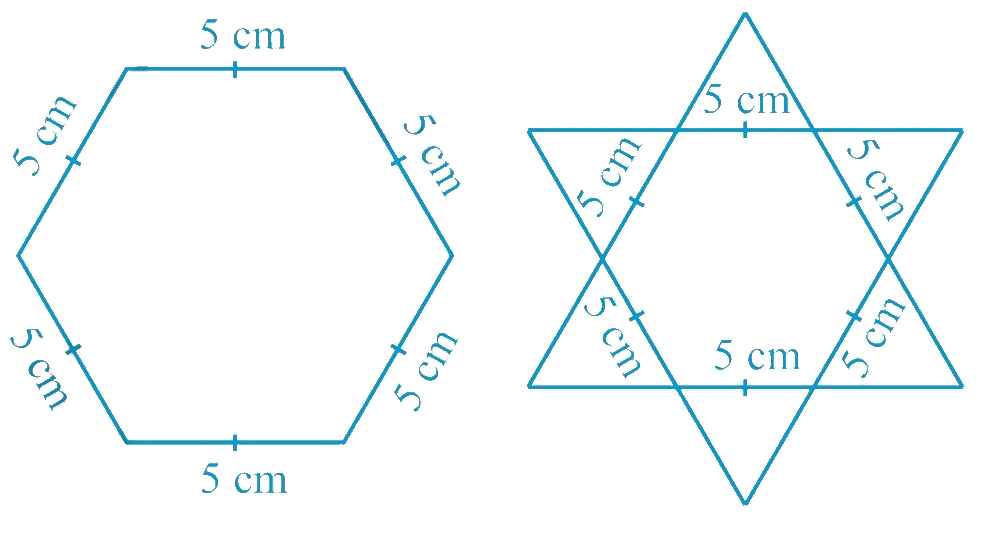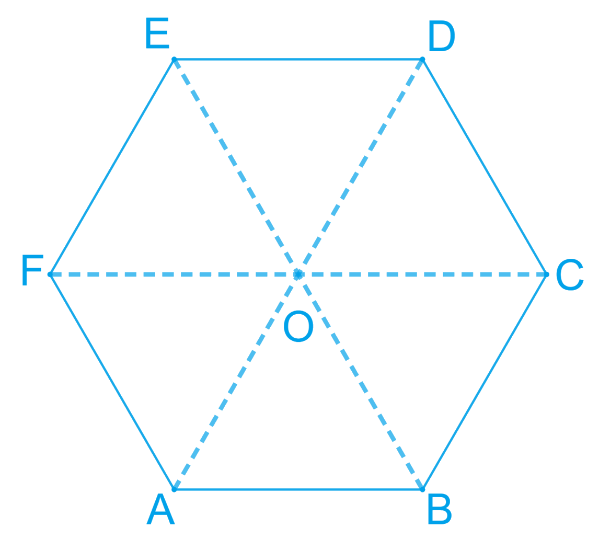# Ex.7.5 Q4 Triangles Solution - NCERT Maths Class 9

Go back to  'Ex.7.5'

## Question

Complete the hexagonal and star shaped rangolies (see the given figures) by filling them with as many equilateral triangles of side $$1\,\rm cm$$ as you can. Count the number of triangles in each case. Which has more triangles?Video Solution
Triangles
Ex 7.5 | Question 4

## Text Solution

Steps:

It can be observed that hexagonal-shaped rangoli has 6 equilateral triangles in it.Area of an equilateral Triangle=$$\frac { \sqrt { 3 } } { 4 } ( \text { side } ) ^ { 2 }$$

\begin{align}\text{Area of } \Delta OAB &= \frac { \sqrt { 3 } } { 4 } ( \text {side} )^{2}\\&= \frac { \sqrt { 3 } } { 4 } ( 25 ) \\ &= \frac { 25 \sqrt { 3 } } { 4 }\; cm ^ { 2 } \end{align}

Area of hexagonal-shaped Rangoli

\begin{align} &= 6 \times \frac { 25 \sqrt { 3 } } { 4 } \\ &= \frac { 75 \sqrt { 3 } } { 2 }\;cm ^ { 2 } \end{align}

Area of equilateral triangle having its side as $$1\,\rm cm$$

\begin{align} & = \frac { \sqrt { 3 } } { 4 } ( 1 ) ^ { 2 } \\ & = \frac { \sqrt { 3 } } { 4 } \; cm ^ { 2 } \end{align}

Number of equilateral triangles of 1 cm side that can be filled in this hexagonal-shaped Rangoli

\begin{align} & = \left( \frac { \frac { 75 \sqrt { 3 } } { 2 } } { \frac { \sqrt { 3 } } { 4 } } \right)\\ & =150 \end{align}

Star-shaped rangoli has $$12$$ equilateral triangles of side $$5\,\rm cm$$ in it.Area of star-shaped rangoli

\begin{align}& = 12 \times \frac { \sqrt { 3 } } { 4 } \times ( 5 ) ^ { 2 } \\ & = 75 \sqrt { 3 } \end{align}

Number of equilateral triangles of 1 cm side that can be filled in this star-shaped rangoli

\begin{align} &= \left( \frac { 75 \sqrt { 3 } } { \frac { \sqrt { 3 } } { 4 } } \right) \\ &= 300 \end{align}

Therefore, star-shaped rangoli has more equilateral triangles in it.

Video Solution
Triangles
Ex 7.5 | Question 4

Learn from the best math teachers and top your exams

• Live one on one classroom and doubt clearing
• Practice worksheets in and after class for conceptual clarity
• Personalized curriculum to keep up with school